Refer to our Texas Go Math Grade 3 Answer Key Pdf to score good marks in the exams. Test yourself by practicing the problems from Texas Go Math Grade 3 Module 16 Assessment Answer Key.

Vocabulary

Choose the best term from the box.

Vocabulary
area
length
unit squares

Question 1.
You can find the __________ of a rectangle by multiplying the number of unit squares in each row by the number of rows. (p. 513)
Explanation:
You can find the area of a rectangle by multiplying the number of unit squares in each row by the number of rows

You can count __________ to find the area of a shape. (p. 513)
Explanation:
You can count Unit Squares to find the area of a shape

Concepts and Skills

Find the area of the figure. Each unit square is 1 square meter. (TEKS 3.6.C, 3.6.D)

Question 3.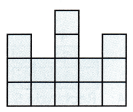___________ square meters
Explanation:
2 x 5 = 10
10 + 4 = 14 square meters

Question 4.___________ square meters
Answer: 3 x 10 = 30
Explanation:
30 square meters

Draw a line to break apart the figure into rectangles. Find the area of the figure. (TEKS 3.6.C, 3.6.D)

Question 5.Rectangle 1: ___________ × ___________ = ___________
Rectangle2: ___________ × ___________ = ___________
___________ + ___________ = ___________ square unitsExplanation:
Rectangle 1:  4 x 4 = 16
Rectangle2: 2 x 5 = 10
16 + 10 = 26 square units

Go Math Module 16 Assessment Grade 3 Pdf Question 6.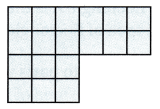Rectangle 1: ___________ × ___________ = ___________
Rectangle2: ___________ × ___________ = ___________
___________ + ___________ = ___________ square unitsExplanation:
Rectangle 1: 4 x 3 = 12
Rectangle2: 2 x 3 = 6
12 + 6 = 18 square units

Fill in the bubble for the correct answer choice.

Question 7.
Peter drew this rectangle. Which equation can be used to find the area? (TEKS 3.6.C)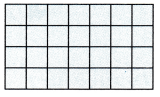(A) 4 × 4 = 16
(B) 7 + 7 + 7 = 21
(C) 4 + 7 + 4 + 7 = 22
(D) 4 × 7 = 28
Explanation:
4 × 7 = 28 equation can be used to find the area

Grade 3 Go Math Module 16 Pdf Question 8.
Alfredo used the Distributive Property to find the area of this rectangle. Which set of multiplication and addition equations could he have used? (TEKS 3.6.C, 36.D)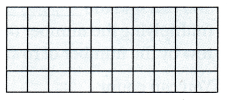(A) 4 + 5 = 9; 4 + 5 = 9; 9 + 9 = 18
(B) 4 + 5 = 9; 4 + 5 = 9; 9 × 9 = 81
(C) 4 × 5 = 20; 4 × 5 = 20; 20 + 20 = 40
(D) 4 × 10 = 40; 4 × 10 = 40; 40 + 40 = 80
Explanation:
4 × 5 = 20; 4 × 5 = 20; 20 + 20 = 40 set of multiplication and addition equations could he have used

Question 9.
Which statement is true about the two rectangles? (TEKS 3.6.C)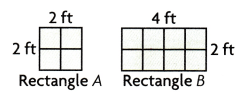(A) The area of Rectangle B is double the area of Rectangle A.
(B) The area of Rectangle A is double the area of Rectangle B.
(C) The area of Rectangle B is half of the area of Rectangle A.
(D) The area of Rectangle A is the same as the area of Rectangle B.
Explanation:
rectangle A = 4
rectangle B = 8
4 x 2 = 8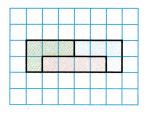(A) $$\frac{1}{2}$$
(B) $$\frac{1}{3}$$
(C) $$\frac{1}{4}$$
(D) $$\frac{1}{6}$$
$$\frac{1}{4}$$ fraction names the area of each part of the divided figure